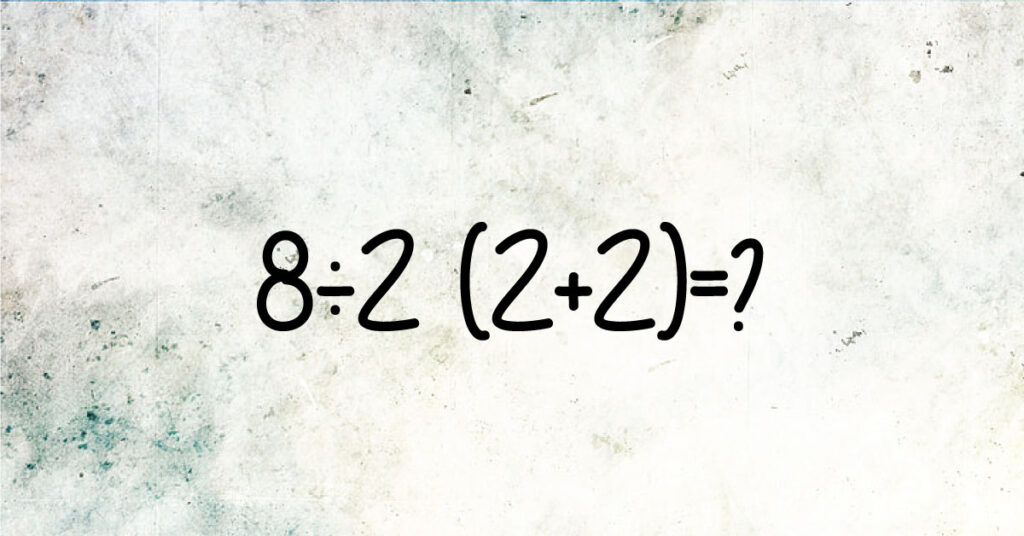# A Math Problem Confused Internet Users – Here Is The Right Answer

## Do you like challenges? Take a look at this one.

A math problem has confused Internet users and they cannot agree on the right answer. Can you find the right answer?

After the Twitter user @pjmdoll shared this math equation, people got confused and divided into two groups. One group thought that the right answer is 16, whereas the other group believed it is 1.

First, they disagreed whether they should apply the principles of Parenthesis, Exponents, Multiplication, Division, Addition, and Subtraction (PEMDAS). When there is a math problem with two or more operations at the same time, the principles of PEMDAS defines the order of what should be calculated first.One of the Internet users said that he had taught math for more than 30 years. He thought that the right answer is 16. As 2 + 2 is four, the problem becomes 8 ÷ 2 (4), or 8 ÷ 2 x 4. The division which is on the left must be done first. Therefore, 4 x 4 = 16.

Nevertheless, some Twitter users applied the principles of Bracket, Of, Division, Multiplication, Addition, and Substruction (BODMAS). The application of BODMAS means removing operators, or brackets, before doing the next operation.

Another Internet user said that the correct answer is one. 8 / 2 (4) = 8 / 8 = 1

Depending on the math principles, many people agreed that it may have two right answers. Those, who tried to solve the math problem with a calculator, said that even the calculator was confused. It can be solved in both ways, depending on which principle you use, PEMDAS or BODMAS.

Mr. Kelly, a math teacher at Ferndale High School, said that the right answer is 16. The users, who think that the right answer is 1, used an old version of the math operations. He added that when there is multiplication or division, we move from left to right, not from right to left. This is the reason why most users got confused. In this case, the division comes first, not the multiplication.

He solved the math problem in this way:

8 / 2 = 4

4 x (2 + 2) or 4 x 4 = 16